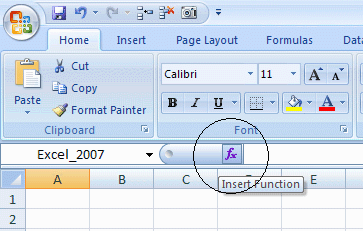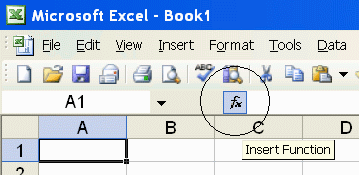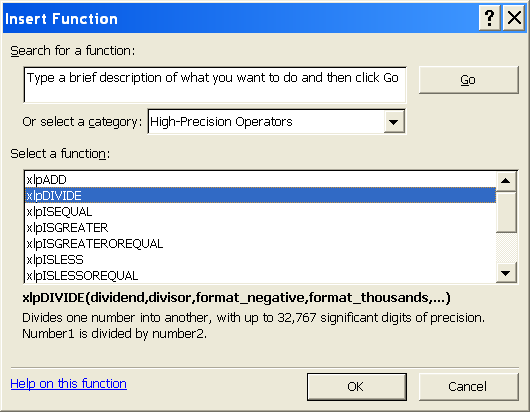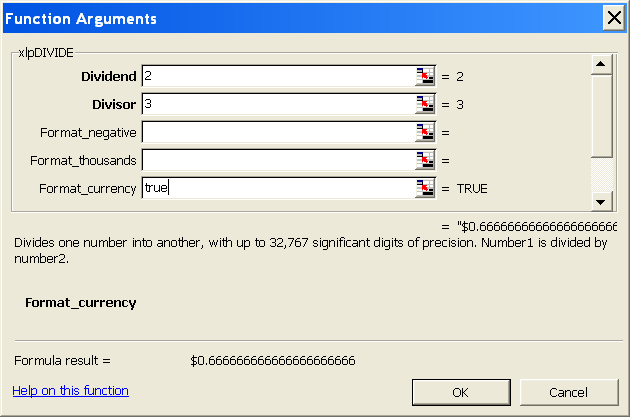PrecisionCalc
xl
Precision
Get Your Numbers Right

xlpROOT

Gets the specified root of a number, with up to 32,767 significant digits of precision.

Syntax

xlpROOT(num,rt,format_negative,format_thousands,format_currency,exponential_notation,
maximum_significant_digits,format_decimal_place)

 num Required. The base number for which a root will be found. Can be positive or negative. Can be a non-integer. rt Required. The root for which num will be found. Can be positive or negative. Can be a non-integer. format_negative Optional. Determines whether negatives are formatted with a leading hyphen ("-"), or parentheses ("()"). Set to 1 to format negatives with a leading hyphen. Set to 2 for parentheses. 1 by default. You can also format negatives with a red font. format_thousands Optional. Determines whether thousands separators are included. Set to TRUE to include thousands separators. FALSE by default. format_currency Optional. Determines whether currency symbol is included. Set to TRUE to include currency symbol. The currency symbol will be added either to the beginning or to the end of the result, whichever is appropriate for the locale. FALSE by default. exponential_notation Optional. Determines whether result is formatted in exponential notation. Set to TRUE to format in exponential notation. FALSE by default. maximum_significant_digits Optional. Determines the maximum number of significant digits to be returned. Default is 100, or the user's custom maximum set in the About box, or the maximum number allowed by the edition of xlPrecision, whichever is less. xlpROOT may be unable to resolve an answer if maximum_significant_digits is the same as, or very close to, the maximum number of significant digits allowed by the xlPrecision edition. If xlpROOT is unable to resolve an answer for this reason, it returns "#NUM!". For best results with xlpROOT, keep maximum_significant_digits at least 5 digits smaller than the maximum number of significant digits allowed by the xlPrecision edition. The default significant digits is always at least 5 digits smaller, so leaving maximum_significant_digits unspecified meets this recommendation. restrict_input_lengths Optional. Returns "#NUM!" if the combined lengths of num and rt are more than half of the maximum number of significant digits allowed by the xlPrecision edition. Set to FALSE to disable this restriction, allowing num and rt to be as long as desired. TRUE by default. xlpROOT may be unable to return a correct answer if the lengths of num and rt add up to more than half of the maximum number of significant digits allowed by your edition of xlPrecision. For example, since the Free Edition allows a maximum of 150 significant digits, restrict_input_lengths would limit num and rt to a combined total of 75 digits. Use restrict_input_lengths with caution; setting restrict_input_lengths to FALSE may cause incorrect results. This limitation applies to the total number of digits in num and rt, not just to the number of significant digits. For example, restrict_input_lengths counts the number "25000" as 5 digits, even though it has only 2 significant digits. restrict_input_lengths is not affected by xlpROOT's maximum_significant_digits argument. For example, if you are using the Free Edition and set xlpROOT's maximum_significant_digits argument to 50, restrict_input_lengths still limits num and rt to a combined total of 75 digits (not 25), because 75 is half of the 150 maximum significant digits allowed by the Free Edition. restrict_input_lengths does not affect how many significant digits xlpROOT can return. If the lengths of num and rt are within the limits, xlpROOT can return as many significant digits as desired. If you are unsure how many significant digits your edition of xlPrecision allows, check Tools | xlPrecision 2.0 | About xlPrecision 2.0, or enter the formula "=xlpSD()" (without the quotes). format_decimal_place Optional. Determines whether to add or remove decimal places as required to return the exact specified number of decimal places.When format_decimal_place causes decimal places to be removed, the remaining decimals are rounded, exactly as Excel's number formatting does. Set format_decimal_place to the number of decimal places desired. For example, set to 3 to specify that the result should have exactly 3 decimal places, so that: "123.4" is formatted as "123.400" "12.3456" is formatted as "12.346" "9.9999" is formatted as "10.000"

Use the Insert Function Dialog to easily enter these arguments:
(available on most editions of xlPrecision, but not recommended with the Free Edition as it causes the Free Edition dialog to appear multiple times)Examples

 Formula Description Result =xlpROOT(8,3) 3 √ 8 2 =xlpROOT(-8,3) 3 √ (-8) -2 =xlpROOT(8,-3) (-3) √ 8 0.5 =xlpROOT(8.5,3) 8.5 √ 3 2.040827550958674035282891 Length of result depends on xlPrecision edition and settings. =xlpROOT(8,3.5) 8 √ 3.5 1.811447328527813343188346 Length of result depends on xlPrecision edition and settings. =xlpROOT(-8,3.5) (-8) √ 3.5 #NUM! If num is negative and rt is not an integer, there is no mathematically correct solution. See explanation below. =xlpROOT(1.23,1.23) 1.23 √ 1.23 1.18329651795922358113431 Length of result depends on xlPrecision edition and settings. =xlpROOT(1.23,-1.23) 1.23 √ -1.23 0.84509671483243561579214 Length of result depends on xlPrecision edition and settings. =xlpROOT(0.9,0.9) 0.9 √ 0.9 0.88952537980417339103166 Length of result depends on xlPrecision edition and settings. =xlpROOT(0.9,-0.9) 0.9 √ -0.9 1.12419501759482938880811 Length of result depends on xlPrecision edition and settings. =xlpROOT("1.23456789123456789",                "9.87654321987654321")To prevent Excel from truncating the numbers to 15 digits, enter them in quotes as shown. 9.87654321987654321 √  1.23456789123456789, without letting Excel truncate to 15 significant digits 1.021564732772948289016690 6451952476219113193988768 Length of result depends on xlPrecision edition and settings. =xlpROOT(-2,-3,2) -3 √ -2, formatted with parentheses if negative (0.793700525984099737375852 81963615413019574666394993) Length of result depends on xlPrecision edition and settings. =xlpROOT(1000000,2,,TRUE) 2 √ 1000000 , formatted with localized thousands separators 1,000  (in the USA) 1.000  (in Germany) 1 000  (in France) =xlpROOT(1000000,2,,TRUE) 2 √ 1000000, formatted with the local currency symbol \$1000  (in the USA) 1000 €  (in Germany) 1000 kr  (in Estonia) 1000Lek  (in Albania) =xlpROOT(1000000,2,,,,TRUE) 2 √ 1000000, formatted in exponential notation 1.0E+3 =xlpROOT("1.002E+200","3.004E+1",,,,TRUE) '3.004E+1' √ '1.002E+200', formatted in exponential notation 4.5479795099043309094479829 760312149818705688843543E+6 Length of result depends on xlPrecision edition and settings. =xlpROOT(A1,A2,,,,,500) =xlpROOT(A1,A2,,,,,500000) =xlpROOT(A1,A2,,,,,A3) To prevent Excel from truncating the numbers in cells A1 & A2 to 15 digits, preformat those cells as "Text" number format, or enter a leading apostrophe. A1 √ A2, with up to 500 (or 500,000, or the number in A3) significant digits of precision. Allows A1, A2, and the return value to each be up to 500 (or 500,000, or the number in A3) significant digits. =xlpROOT(8,3,,,,,,,3) 3 √ 8, formatted to 3 decimal places. 2.000 (padded with zeros to meet the specified number of decimal places) =xlpROOT(7,3,,,,,,,3) 3 √ 7, formatted to 3 decimal places. 1.913 (rounded to fit the specified number of decimal places) =xlpROOT(-1000000000,3,2,TRUE,TRUE,,500,,2) 3 √ -1000000000, formatted with: parentheses if negative localized thousands separators local currency symbol up to 500 significant digits of precision 2 decimal places (\$1,000.00)  (in the USA) (1.000,00 €)  (in Germany) (1 000,00 €)  (in France) (1 000.00 kr)  (in Estonia) (1.000,00Lek)  (in Albania)

Remarks

• If num is negative and rt is not an integer, xlpROOT will correctly return "#NUM!". The reason "#NUM!" is returned is because there is no correct solution. If num is negative and rt is not an integer, there is no solution which can be raised to the power of rt to resolve to num. If no solution can be raised to the power of rt to resolve to num, then by definition there is no correct solution. For example:

=xlpROOT(-3, 2.5)

This formula correctly returns "#NUM!", because there is no solution which can be raised to the power of 2.5 to resolve to -3. Thus, by definition, -3 does not have a 2.5 root.

• xlPrecision results are returned as text that look like numbers, not as values that Excel recognizes as numbers. This is because Excel would truncate the results to 15 significant digits if it recognized them as numbers.

• Some arguments are ignored in some editions of xlPrecison. See the Buy page for details.

• maximum_significant_digits is ignored if it is higher than the maximum significant digits allowed by the edition of xlPrecision.

• Use maximum_significant_digits to increase calculation speed where desired. The lower the number used, the faster the calculation.

• num and rt can accept both numbers and text.

• num and rt can accept text formatted with the local currency symbol and thousands separators, and negatives can be formatted with either a leading hyphen or parentheses. They also can accept numbers formatted in scientific notation.

• num and rt can accept text up to 32,767 characters long.

• You can use the results of xlPrecision functions as the operands in other xlPrecision formulas without losing any precision, but using them as operands in Excel's arithmetic functions will truncate them to 15 significant digits.

• If the return value is so large that it has more than 32,767 characters to the left of the decimal, then xlPrecision is of course unable to return a correct value and instead returns "#VALUE!". To return that data successfully, set exponential_notation to TRUE to return the value in exponential notation.

• 32,767 digits to the left of the decimal is a vastly larger number than Excel can return without xlPrecision. Excel itself can only return or recognize a number with no more than 308 digits to the left of the decimal.

• Decimal symbols, thousands separators, and currency symbols are all localized. This means that an xlPrecision formula that returns "\$1,234,567.89" in the USA will return "1.234.567,89 €" in Germany, "1 234 567,89 €" in France, "1 234 567.89 kr" in Estonia, and "1.234.567,89Lek" in Albania.

• The 32,767 SD edition can only provide a maximum of 32,767 total characters, including all formatting characters such as decimal, leading hyphen or parentheses for negatives, and thousands separators. As a result, it can only return the maximum 32,767 significant digits when the result is an unformatted positive integer. This is due to Excel's limitation of 32,767 characters in a cell. In all cases, the 32,767 SD edition will give you as many significant digits as possible with the formatting you have chosen.

• Depending on how many significant digits the edition of xlPrecision provides, the result may be too long to conveniently view. You can view the full result by right-clicking the cell and choosing Format Cells | Alignment | Wrap Text, and widening the column to the width of the screen. An easy way to view the full result without changing column widths or wrapping text is to right-click the cell, choose Copy, and then paste into Notepad or a word processor.